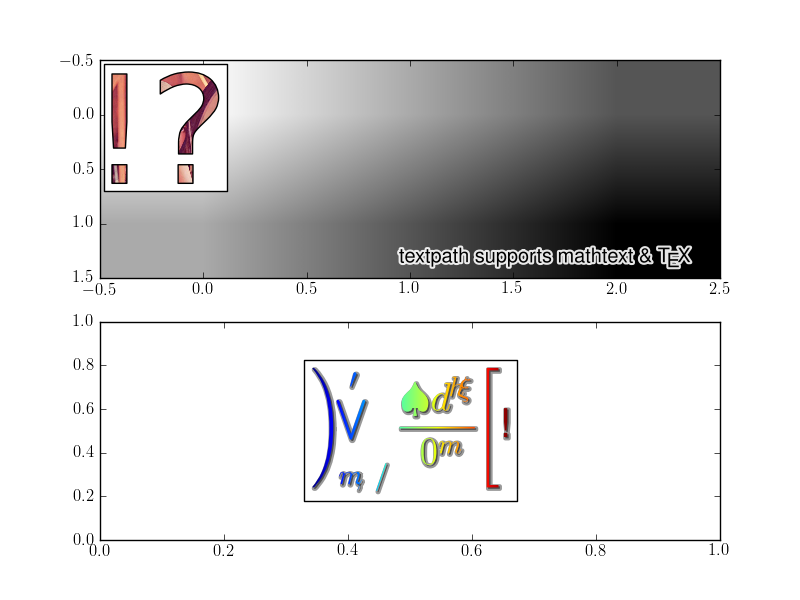# Incorrect output from demo_text_path.py on mac

Hi,

I am running the svn version of matplotlib on a mac (OSX 10.6 with latest macports), and there is a problem with the output from demo_text_path.py. It does give the correct output on linux, so I assume the problem is either with the installed fonts on the mac, or with the mathtext code (in particular the tables in _mathtext_data.py). From the output, you can see that the text is correct in the upper subplot, but in the lower one, where is uses mathematical symbols, it is garbled. I get the same output for different backends (I have checked with macosx, GTKAgg and pdf).

If I can help to figure out the problem, please let me know,

regards,

tcbThanks for the report.
And I wonder if you can provide us some more details of your
configuration and do some more tests.

1) You're not using usetex mode, correct?

2) Does your mathtext work fine otherwise? I mean, with the ordinary
text command, not the textpath example.

Regards,

-JJ

···

On Mon, Nov 2, 2009 at 2:45 PM, tcb <thecolourblue+m@...149...> wrote:

Hi,

I am running the svn version of matplotlib on a mac (OSX 10.6 with latest
macports), and there is a problem with the output from demo_text_path.py. It
does give the correct output on linux, so I assume the problem is either
with the installed fonts on the mac, or with the mathtext code (in
particular the tables in _mathtext_data.py). From the output, you can see
that the text is correct in the upper subplot, but in the lower one, where
is uses mathematical symbols, it is garbled. I get the same output for
different backends (I have checked with macosx, GTKAgg and pdf).

If I can help to figure out the problem, please let me know,

regards,

tcb

------------------------------------------------------------------------------
Come build with us! The BlackBerry(R) Developer Conference in SF, CA
is the only developer event you need to attend this year. Jumpstart your
developing skills, take BlackBerry mobile applications to market and stay